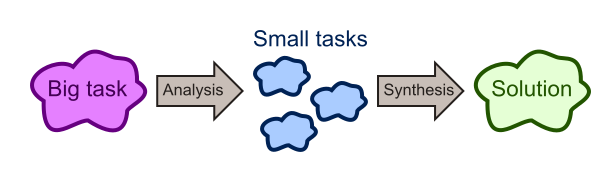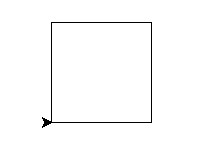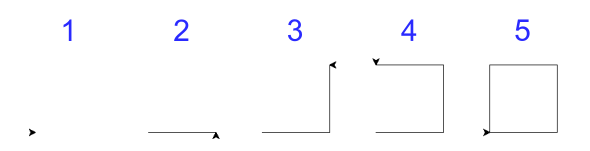# Decomposition

Martin McBride, 2016-12-18
Tags decomposition turtle graphics
Categories algorithms computational thinking

Decomposing a problem simply means breaking it down into a set of smaller problems which can be solved more easily. It is a useful way of tackling any task or problem which seems to difficult to solve in one go. Here are some examples.

## Baking a cake

A recipe is a good example of decomposition. It provides step by step instructions, for example to make a Victoria sponge:

• Cream the butter and sugar together, in a bowl
• Beat in the eggs
• Add vanilla
• Fold in the flour
• Place the mixture in a cake tin
• Bake in a medium oven for 25 minutesEven if you have never baked a cake before, and don't have a clue how to do it, when it is broken down into steps like this it becomes much easier. Each one of the steps looks quite straightforward, and if you follow them carefully you will have some kind of cake at the end.

We sometimes have to decompose a problem in more than one way in order to fully understand it. For example the recipe above needs an ingredients list, giving the weight of butter, sugar and flour to use, and how many eggs. It might also give us a list of the equipment we need (bowl, weighing scales, mixer, cake tin). So in this case we analyse the task of baking a cake, breaking down:

• What it is made of (the ingredients list)
• How it is made (the recipe)
• The tools we need (bowls, scales etc)

Analysing the cake in this way also allows us to work out:

• How long it will take to make
• How much it will cost
• How many calories it will have

## Writing an essay

As another example of decomposition, think about what you might do if you have a homework essay to write.

You could just dive in and start writing, but you might find that isn't the best idea. It is usually better to plan first, to decide what are the key points or arguments you want to get across. Then choose examples that best illustrate your points. When you put this all together, you will have a clear and coherent essay.

For example, here is what I did when writing the page you are reading right now. First I decided on the main points:

• Decomposition is a way of solving complex problems
• It works by breaking down a difficult problem into a collection of smaller, easier problems
• This process is called analysis (that is what we are doing at the moment)
• When you have solved all the parts, you bring them all together into a complete solution - this is called synthesis

You might want to make a list, or even a mind map at this point.

So next I decided what examples I wanted to use - baking a cake, writing an essay, and drawing a square using turtle graphics (the next section).

Decomposition involves analysis - looking at the task or problem and deciding how to break it down. In the example of baking a cake, the analysis was done for us by the author of the cookbook. But when you are writing an essay, you must do the analysis yourself.After the analysis stage, you must bring all the parts together when you finally write your essay - the process of synthesis. You have decided what the main sections will be, and you have a list of bullet points to include, but you still have to write the words

## Turtle graphics

Turtle graphics provide a simple, graphical programming environment. This allows us to explore computational thinking topics without getting bogged down in complicated code. This example will be extended to look at the other topics - recognising patterns, abstraction and algorithms.

Here is a square drawn with the turtle:As before, we want to decompose the task of drawing the square. Here it is in stages:1. We start with the turtle at its starting position, pointing to the left. The arrow represents the turtle and show its direction.
2. We move the turtle forward by 100 pixels to draw the first side, then turn it left by 90 degrees.
3. We move the turtle forward by 100 pixels to draw the second side, then turn it left by 90 degrees.
4. We move the turtle forward by 100 pixels to draw the third side, then turn it left by 90 degrees.
5. We move the turtle forward by 100 pixels to complete the shape. We also turn it left by 90 degrees, so it ends up exactly where it started

This analysis shows us how to draw the square - by moving and turning 4 times. Here is the Python code to draw it (if you are using a different Turtle system, it should be easy to translate the commands):

import turtle

turtle.forward(100)
turtle.left(90)
turtle.forward(100)
turtle.left(90)
turtle.forward(100)
turtle.left(90)
turtle.forward(100)
turtle.left(90)


Copyright (c) Axlesoft Ltd 2021